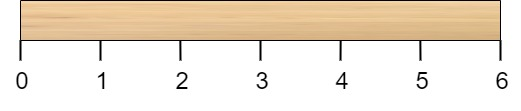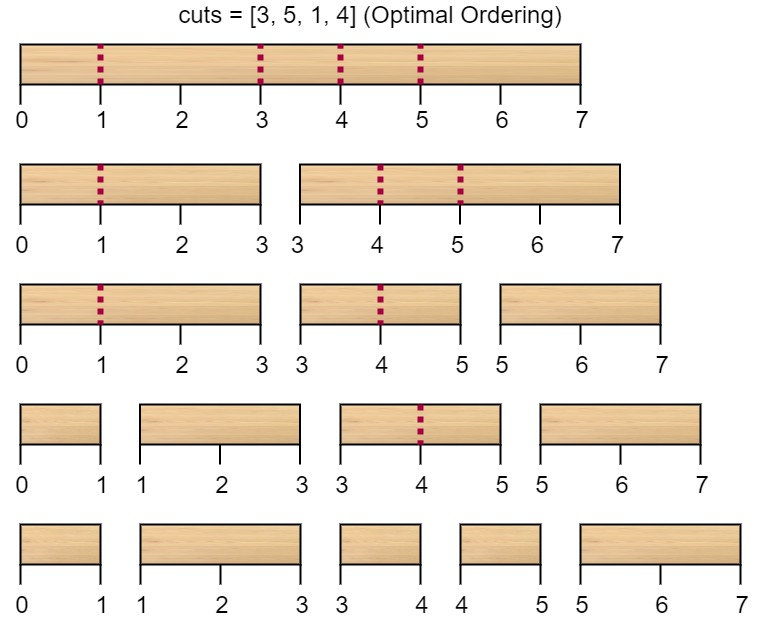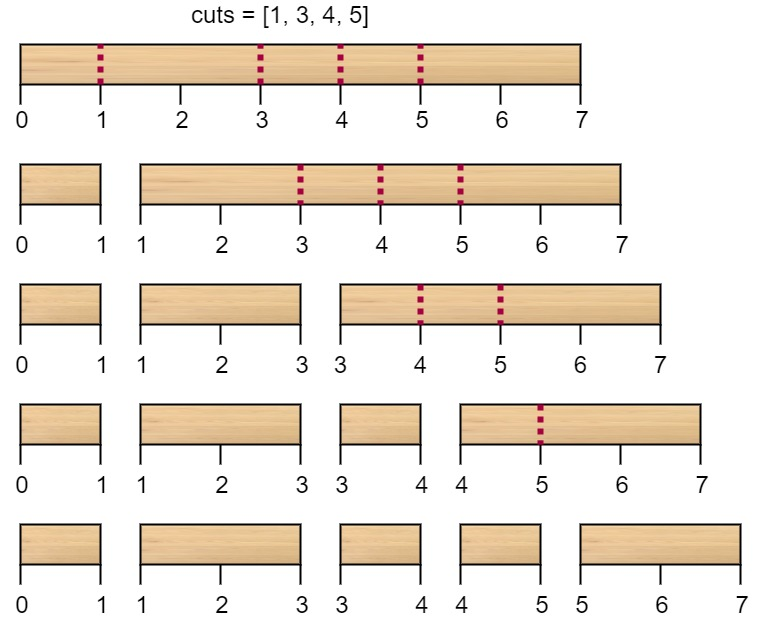# 切棍子的最小成本

## 1547. 切棍子的最小成本 (Hard)```输入：n = 7, cuts = [1,3,4,5]```

```输入：n = 9, cuts = [5,6,1,4,2]

• `2 <= n <= 10^6`
• `1 <= cuts.length <= min(n - 1, 100)`
• `1 <= cuts[i] <= n - 1`
• `cuts` 数组中的所有整数都 互不相同

[数组] [动态规划]